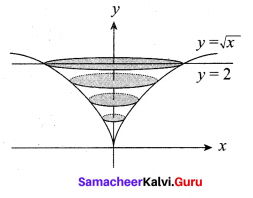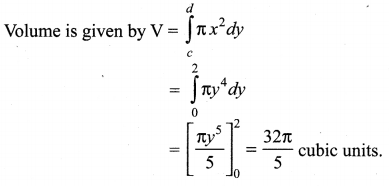Tamilnadu Samacheer Kalvi 12th Maths Solutions Chapter 9 Applications of Integration Ex 9.9

Question 1.
Find, by integration, the volume of the solid generated by revolving about the x-axis, the region enclosed by y = 2x2, y = 0 and x = 1.
Solution: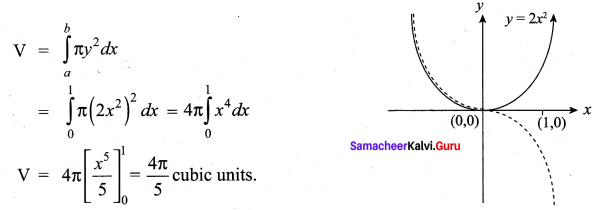Question 2.
Find, by integration, the volume of the solid generated by revolving about the x-axis, the region enclosed by y = e-2x y = 0, x = 0 and x = 1.
Solution: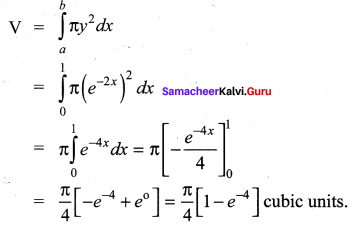Question 3.
Find, by integration, the volume of the solid generated by revolving about the y-axis, the region enclosed by x2 = 1 + y and y = 3.
Solution: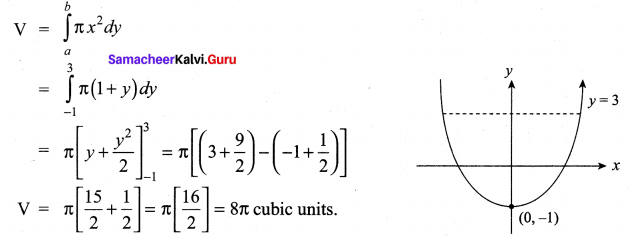Question 4.
The region enclosed between the graphs of y = x and y = x2 is denoted by R, Find the volume generated when R is rotated through 360° about x – axis.
Solution:
To find points of intersection, solving y = x2 and y = x, we get (0, 0) and (1, 1)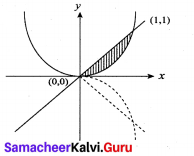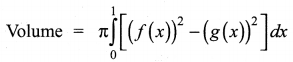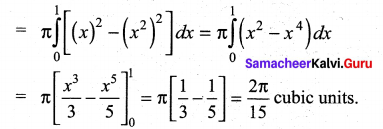Question 5.
Find, by integration, the volume of the container which is in the shape of a right circular conical frustum as shown in the Figure.
Solution: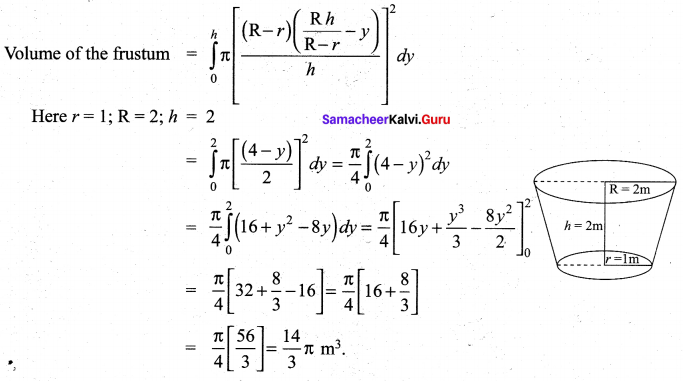Question 6.
A watermelon has an ellipsoid shape which can be obtained by revolving an ellipse with major-axis 20 cm and minor-axis 10 cm about its major-axis. Find its volume using integration.
Solution:
From the given data a = 10 cm and b = 5 cm
Equation of the Ellipse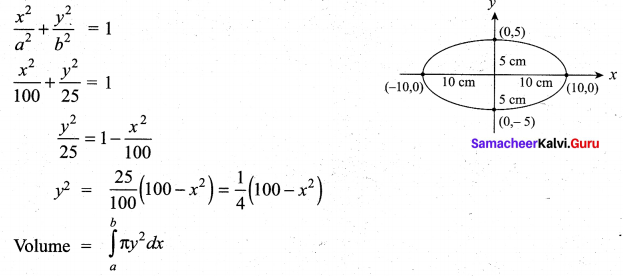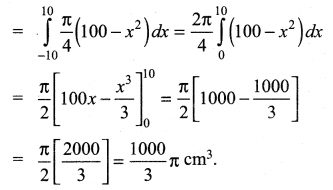Samacheer Kalvi 12th Maths Solutions Chapter 9 Applications of Integration Ex 9.9 Additional Questions

Question 1.
Find the volume of the solid that results when the ellipse $$\frac{x^{2}}{a^{2}}+\frac{y^{2}}{b^{2}}=1$$ (a > b > 0) is revolved about the minor axis.
Solution:
Volume of the solid is obtained by revolving the right side of the curve $$\frac{x^{2}}{a^{2}}+\frac{y^{2}}{b^{2}}=1$$ about the y-axis.
Limits for y is obtained by putting x = 0 ⇒ y2 = b2 ⇒ y = ±b.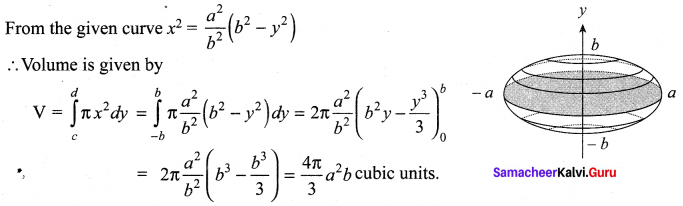Question 2.
Find the volume of the solid generated when the region enclosed by y = $$\sqrt{x}$$, y = 2 and x = 0 is revolved about the y – axis.
Solution:
Since the solid is generated by revolving about the y-axis, rewrite y = $$\sqrt{x}$$ as x = y2.
Taking the limits for y, y = 0 and y = 2 (Putting x = 0 in x = y2, we get y = 0)